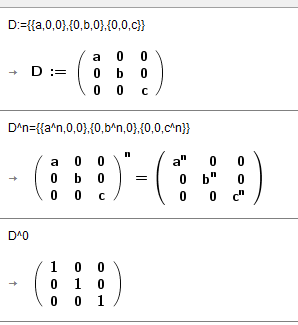# Zero to the zeroth power

Hodorious shared this problem 7 years ago
Not a Problem

GeoGebra evaluates 0^0 to 1, which is not correct, or at least there is no consensus on this. Moreover, when you try to raise zero to a negative power, the program just outputs infinity. 0^0 is evaluated to 1 in the Algebra and Spreadsheet view. In CAS, 0^0 just returns a question mark, so there's also a lack of consistency.

Using GeoGebra 5.0.266.01

Yes, that's intended1

In CAS:

0^0 returns a question markIsDefined(0^0) returns true

Why?1

IsDefined() isn't implemented in the CAS (although by coincidence it will be for next release :) )11
Files: 0^0.jpg11

Of course, I know that. However, in reality it is just a form of notation.

Instead of Sum(Sequence(x^i/i!,i,0,n)), it is sufficient to write

1+Sum(Sequence(x^i/i!,i,1,n)), or Sum(Sequence(If(i>0,x^i,1)/i!,i,0,n)).

To define 0^0 is useful for programmers but it might not be suitable for

teachers of mathematics. GeoGebra seems to have sometimes forgotten about high-school mathematics, the pupils and their teachers. I looked into several textbooks that I have at hand and each of them deals with the topic of Zero and Negative Integer Exponents, but neither defines the expression 0^0.

Here is the list of textbooks:

Aufmann, R. N., Lockwood, J. S.: Introductory Algebra. An Applied Approach, 2010.

Aufmann, R. N., Barker, V. C., Nation, R. D.: College Algebra and Trigonometry, 2011.

Axler, S.: Algebra and Trigonometry, 2012.

Baratto, S., Bergman, B., Hutchinson, D.: Elementary and Intermediate Algebra, 2010.

Barnett, R. A., Ziegler, M. R., Byleen, K. E., Sobecki, D.: College Algebra, 2011.

Barnett, R. A., Ziegler, M. R., Byleen, K. E.: Colege Mathematics for Business, Econonics, Life Sciences, and Social Sciences, 2015.

Barnett, R. A., Ziegler, M. R., Byleen, K. E.: Precalculus, Functions and Graphs, 2001.

Barnett, R. A., Ziegler, M. R., Byleen, K. E., Sobecki, D.: Precalculus: Graphs and Models, 2009.

Beecher, J. A., Penna, J. A., Bittinger, M. L.: Algebra and Trigonometry, 2012.

Beleznay, F.: Mathematics for the IB Diploma, 2016.

Bello, I.: Introductory Algebra, A Real World Approach, 2009.

Bettinger, A. K., Englund, J. A.: Algebra and Trigonometry, 1963.

Bittinger, M. L., Ellenbogen D. J., Johnson, B. L.: Elementary and Intermediate Algebra: Graphs and Models, 2012.

Blitzer, R.: College Algebra, 2004.

Brown, S. A., Breunlin, R. J., Wiltjer, M. H.: UCSMP Algebra, 2004.

Cohen, D.: Precalculus, A Problem-Oriented Approach, 2005.

Crisler, N., Simundza, G.: Modeling with Mathematics, A Bridge to Algebra II, 2012.

Dugopolski, M.: Elementary and Intermediate Algebra, 2012.

Gantert, A. X.: Algebra 2 and Trigonometry, 2009.

Martin-Gay, K. E.: Algebra, A Combined Approach, 2012.

Martin-Gay, K. E., Greene, M.: Intermediate Algebra, A Graphing Approach, 2014.

McGraw-Hill authors: IMPACT Mathematics: Algebra and More, Course 3, Student Edition, 2005.

Gloag, A., Gloag, A., Kramer, M., Introductory Algebra, CK-12 Foundation, 2012.

Gustafson, R. D., Hughes, J. D.: College Algebra, 2013.

Holliday, B., Luchin, B., Cuevas, G. J., Carter, J. A., Marks, D., Day, R., Casey,R. M., Hayek, L. M.: Algebra 2, 2008.

Schultz, J. E., Ellis, W., Hollowell, K. A., Kennedy, P. A.: Algebra 2, 2004.

Hornsby, J., Lial, M. L. Rockswold, G.: A Graphical Approach to College Algebra, 2015.

Kaufmann, J. E., Schwitters, K. L.: Algebra for College Students, 2011.

Kaufmann, J. E., Schwitters, K. L.: College Algebra, 2004.

Kime, L. A., Clark, J., Michael, B. K.: Explorations in College Algebra, 2007.

Larson, R., Hostetler, R., Kelley, P. M.: Intermediate Algebra, 2008.

Larson, R., Falvo, D. C.: Algebra and Trigonometry, 2011.

Larson, R., Hodgkins, A. V.: College Algebra with Applications for Business and the Life Sciences, 2009.

Larson, R., Boswell, L., Kanold, T. D., Sti , L., Algebra 1. Concepts and Skills, 2004.

Larson, R., Boswell, L., Kanold, T. D., Sti , L., Algebra 1, 2004.

Lehmann, J.: Elementary Algebra, Graphs and Authentic Applications, 2015.

Malloy, C. E., Molix-Bailey, R. J., Price, J., Willard, T.: Pre-Algebra, 2008.

McCallum, W. G., Connally, E., Hughes-Hallet, D., et al.: Algebra, Form and Function, 2010.

McCune, S. L.: Algebra I, Review and Workbook, 2019.

Murdock, J., Kamischke, E., Kamischke, E.: Discovering Algebra, An Invstigative Approach, 2007.

Narasimhan, R.: Precalculus, Building Concepts and Connections, 2009.

Coene, C., LeBlanc, D., Edwards, B., Sherk, R. et al.: Foundations for College Mathematics, 2009.

Stewart, J., Redlin, L., Watson, S.: Precalculus, Mathematics for Calculus, 2009.

Sultan, A., Atrzt, A. F.: The Mathematics That Every Secondary Scholl Math TeacherNeeds to Know, 2018.

Swokowksi, E. W., Cole, J. A.: Algebra and Trigonometry with Analytic Geometry, 2008.

Tussy, A. S., Gustafson, R. D.: Developmental Mathematics for College Students, 2006.

Tussy, A. S., Gustafson, R. D., Koenig, D. R.: Intermdiate Algebra, 2011.

Tussy, A. S., Gustafson, R. D.: Intermdiate Algebra, 2013.

Coburn, J. W., Herdlick, J. D.: College Algebra, Graphs and Models, 2012.

Charles, R. I., Hall, B., Kennedy, D., Bellman, A. E. et al.: Algebra 1, Common Core, 2015.1

```neither defines the expression 0^0.
```
So will you write to the authors so they can correct their next editions?

1 used to be considered prime, now it's not. It's just a matter of convention, same with 0^0. I used to think the same as you but it's simply more pragmatic to define 0^0=11

otro ejemplo. A mí me gusta que funcione

aunque a o b o c sean cero